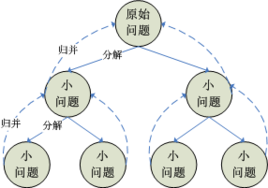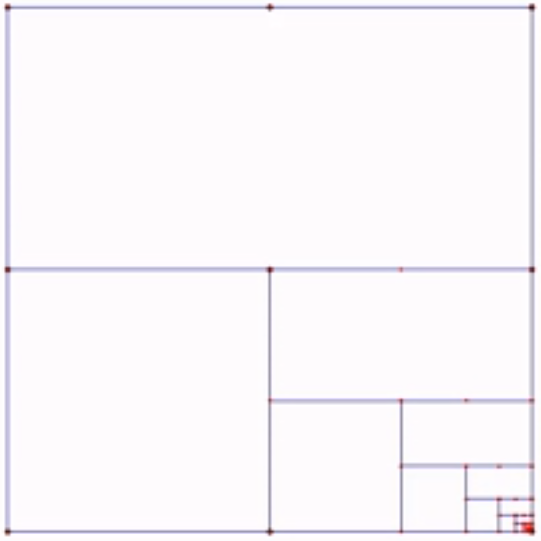### 【算法】分治四步走

1 ) 分解：将原问题分解为若干个规模较小，相互独立，与原问题形式相同的子问题
2 ) 解决：若子问题规模较小而容易被解决则直接解，否则递归地解各个子问题
3 ) 合并：将各个子问题的解合并为原问题的解。

# 分治四步走

1. 明确分解策略：明确大问题通过怎样的分解策略一步步分解为最终的小问题，之后我们需要根据分解策略明确函数的功能
比如说，我们的分解策略如果是折半分解，那么我们的函数就需要有范围域来确定分解范围；如果是递减分解，那么我们的函数需要有计数，来记录递减分解结果。

比如说，快速排序的大的问题可以分解为就是将n个元素摆到正确位置，汉诺塔的大的问题就是将n个圆盘由下而上摆到正确位置。

2. 寻找最小问题：最小问题也就是大问题的最简化版本，问题的起始状态，最小子问题即是出口。

3. 解决次小问题：使用分解策略将大问题分解为次小的问题。次小问题也就是介于最小问题与大问题之间的问题，比最小问题稍稍大那么一点，这使得次小问题具有解决大问题的通用性，即 可以通过次小问题找到大问题的通解。由次小问题得到解决方法。

比如说，快速排序的次小问题就是将一个元素摆到正确位置，汉诺塔的次小问题就是将一个最下面的圆盘摆到正确位置。

4. 合并次小问题：这个按照问题需要进行添加。## 明确分解策略

``````//找出一个数组中的最大值
// 明确分解策略：大问题=从n个元素中找到最大的数字并返回，折半分解，小问题=从2个元素比较大小找到最大数字并返回。
int f(int[] nums, int l, int r) {

}``````

## 寻找最小问题（初始条件）• 必须有一个明确的结束条件。因为分治就是有“分”“并”，所以必须又有一个明确的点，到了这个点，就不用“分解下去”，而是开始“合并”。

``````// 明确分解策略：大问题=从n个元素中找到最大的数字并返回，折半分解，小问题=从2个元素比较大小找到最大数字并返回。
int f(int[] nums, int l, int r) {

// 寻找最小问题：最小问题即是只有一个元素的时候
if (l >= r) {
return nums[l];
}
}``````

## 解决次小问题

• 最小问题：分解至只剩一个元素，l>=r，f(l)即为最大值
• 分解策略：折半分解，f(nums, l, r) → f(nums, l, (l+r)/2) , f(nums, (l+r)/2+1, r)

• 分：将f(nums, l, r) → f(nums, l, (l+r)/2) , f(nums, (l+r)/2+1, r)了。这样，问题就由n缩小为了n/2，我们只需要找到这n/2个元素的最大值即可。就这样慢慢从f(n),f(n/2)“分”到f(1)。
• 并：这样就可以从1，一步一步“并”到n/2,n…
``````// 明确分解策略：大问题=从n个元素中找到最大的数字并返回，折半分解，小问题=从2个元素比较大小找到最大数字并返回。
int f(int[] nums, int l, int r) {

// 寻找最小问题：最小问题即是只有一个元素的时候
if (l >= r) {
return nums[l];
}

// 使用分解策略
int lMax = f(nums, l, (l+r)/2);
int rMax = f(nums, (l+r)/2+1, r);
}``````

``````// 明确分解策略：大问题=从n个元素中找到最大的数字并返回，折半分解，小问题=从2个元素比较大小找到最大数字并返回。
int f(int[] nums, int l, int r) {

// 寻找最小问题：最小问题即是只有一个元素的时候
if (l >= r) {
return nums[l];
}

// 使用分解策略
int lMax = f(nums, l, (l+r)/2);
int rMax = f(nums, (l+r)/2+1, r);

// 解决次小问题：比较两个元素得到最大的数字
return lMax > rMax ? lMax : rMax;
}``````

## 合并次小问题

f(1)只有一个元素，可以得到一个确定的值
f(2)比较f(1)的值，也能确定了
f(4)比较f(2)的值，也能确定下来了

f(n/2)
f(n)比较f(n/2)也能确定下来了

# 实例

## 最大数

``````// 明确分解策略：大问题=从n个元素中找到最大的数字并返回，折半分解，小问题=从2个元素比较大小找到最大数字并返回。
int f(int[] nums, int l, int r) {

// 寻找最小问题：最小问题即是只有一个元素的时候
if (l >= r) {
return nums[l];
}

// 使用分解策略
int lMax = f(nums, l, (l+r)/2);
int rMax = f(nums, (l+r)/2+1, r);

// 解决次小问题：比较两个元素得到最大的数字
return lMax > rMax ? lMax : rMax;
}``````

## 汉诺塔

1 ) 如果是有一个盘，A->C

2 ) 先把 最上面的盘A->B
3 ) 把最下边的盘A->C
4 ) 把B塔的所有盘 从 B->C

``````package com.atguigu.dac;

public class Hanoitower {

public static void main(String[] args) {
hanoiTower(10, 'A', 'B', 'C');
}

//汉诺塔的移动的方法
//使用分治算法
// 明确分解策略：我们的问题是有n个盘子，可是如果是n个盘子的话我们不会分，不知道结果；如果盘子数量为1、2、3就好了，所以我们按盘子数依次减一分解
public static void hanoiTower(int num, char a, char b, char c) {
// 寻找最小问题：只有一个盘
//如果只有一个盘
if(num == 1) {
System.out.println("第1个盘从 " + a + "->" + c);
} else {
// 解决次小问题：由于我们是按盘子数-1来进行分解的，所以次小问题是一个盘子和n-1个盘子的汉诺塔，将一个最下面的盘子摆放到正确的位置
//如果我们有 n >= 2 情况，我们总是可以看做是两个盘 1.最下边的一个盘 2. 上面的所有盘
//1. 先把 最上面的所有盘 A->B， 移动过程会使用到 c
hanoiTower(num - 1, a, c, b);
//2. 把最下边的盘 A->C
System.out.println("第" + num + "个盘从 " + a + "->" + c);
//3. 把B塔的所有盘 从 B->C , 移动过程使用到 a塔
hanoiTower(num - 1, b, a, c);
}
}
}``````
Tags: